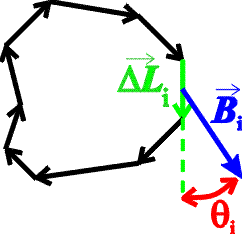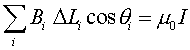Ampere's law Ampere's law is the magnetic equivalent of Gauss's law. It is different in that it refers to a closed loop and the surface enclosed by it (rather than a closed surface and the volume enclosed by it, as is the case with Gauss's Law). Consider a closed loop, not necessarily a circle, which is broken into small elements of length DLi with a magnetic field Bi at each element.The sum over elements of the component of the magnetic field along the direction of the element, times the element length, is proportional to the current I that passes through the loop.This is Ampere's law. For the case of a wire, the loop can be a circle drawn around the wire, and since the field is always tangent to the circle, cosq = 1. The circumference of the circle of radius r is 2pr, therefore Ampere's law becomes:which is the expression for the magnetic field of a wire given before. Ampere's law will also allow us to calculate the magnetic field for a solenoid.